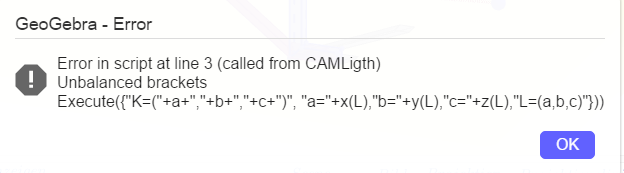hawe shared this problem 1 year ago
Not a Problem

CheckBox POS Ligth (POS CAM)

the skript has done work - for a while, but

If(CAMLigth,

Execute({"L=("+a+","+b+","+c+")", "a="+x(K),"b="+y(K),"c="+z(K),"K=(a,b,c)"}),

Execute({"K=("+a+","+b+","+c+")", "a="+x(L),"b="+y(L),"c="+z(L),"L=(a,b,c)"}))

If(CAMLigth,SetCaption(CAMLigth,"POS Kamera"),SetCaption(CAMLigth,"POS Licht"))What should be wrong to that script - when I put the lists in variables it is acepted.1

({............................ })) I think last ) must be deleted1

this is closing if clause

as i said this script has been working, but suddenly throwing an error1

just count the brackets and remove the last one as mathmagic wrote. You are closing one more than you opened, so it can't work.

chris1

see working version of script

https://www.geogebra.org/cl...1

el script que trabaja tiene dos lineas

posiblemente has copiado y pegado como si tuviera cuatro lineas. elimina los saltos de linea al final de la primera y la segunda para volver a tener dos lineas